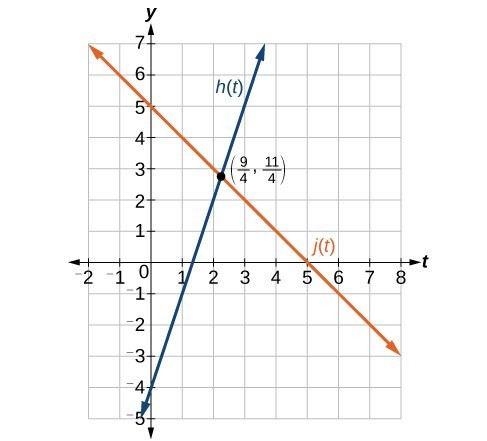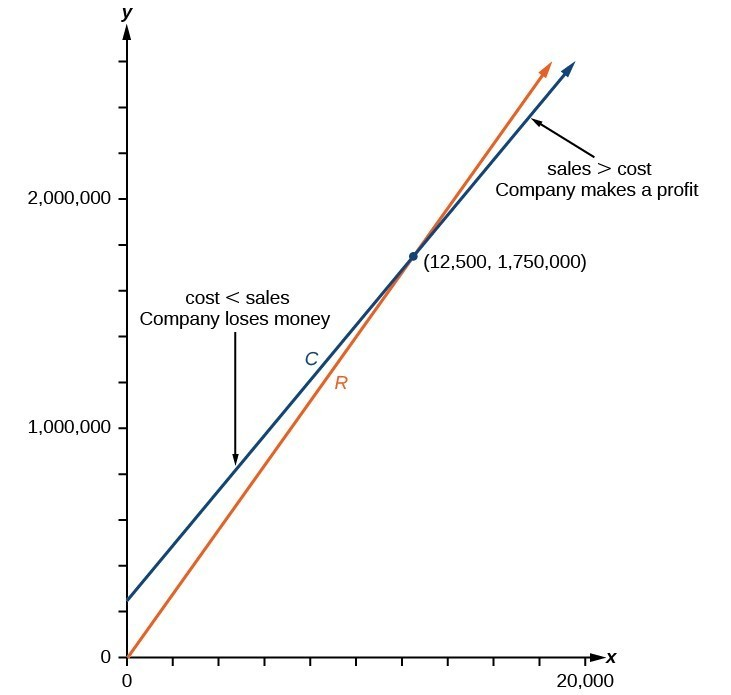## Solve a system of linear equations

A system of linear equations includes two or more linear equations. The graphs of two lines will intersect at a single point if they are not parallel. Two parallel lines can also intersect if they are coincident, which means they are the same line and they intersect at every point. For two lines that are not parallel, the single point of intersection will satisfy both equations and therefore represent the solution to the system.

To find this point when the equations are given as functions, we can solve for an input value so that $f\left(x\right)=g\left(x\right)$. In other words, we can set the formulas for the lines equal to one another, and solve for the input that satisfies the equation.

### Example 12: Finding a Point of Intersection Algebraically

Find the point of intersection of the lines $h\left(t\right)=3t - 4$ and $j\left(t\right)=5-t$.

### Solution

Set $h\left(t\right)=j\left(t\right)$.

$\begin{cases}3t - 4=5-t\hfill \\ \text{ }4t=9\hfill \\ \text{ }t=\frac{9}{4}\hfill \end{cases}$

This tells us the lines intersect when the input is $\frac{9}{4}$.

We can then find the output value of the intersection point by evaluating either function at this input.

$\begin{cases}j\left(\frac{9}{4}\right)=5-\frac{9}{4}\hfill \\ \text{ }=\frac{11}{4}\hfill \end{cases}$

These lines intersect at the point $\left(\frac{9}{4},\frac{11}{4}\right)$.

### Analysis of the Solution

Looking at Figure 24, this result seems reasonable.Figure 24

### Q & A

If we were asked to find the point of intersection of two distinct parallel lines, should something in the solution process alert us to the fact that there are no solutions?

Yes. After setting the two equations equal to one another, the result would be the contradiction “0 = non-zero real number”.

### Try It 8

Using the graph in the Analysis of the Solution for Example 12, identify the following for the function $j\left(t\right)$:

a. y-intercept

b. x-intercept(s)

c. slope

d. Is $j\left(t\right)$ parallel or perpendicular to $h\left(t\right)$ (or neither)?

e. Is $j\left(t\right)$ an increasing or decreasing function (or neither)?

f. Write a transformation description for $j\left(t\right)$ from the identity toolkit function $f\left(x\right)=x$.

Solution

A company sells sports helmets. The company incurs a one-time fixed cost for $250,000. Each helmet costs$120 to produce, and sells for $140. 1. Find the cost function, C, to produce x helmets, in dollars. 2. Find the revenue function, R, from the sales of x helmets, in dollars. 3. Find the break-even point, the point of intersection of the two graphs and R. ### Solution 1. The cost function in the sum of the fixed cost,$125,000, and the variable cost, \$120 per helmet.
$C\left(x\right)=120x+250,000$
2. The revenue function is the total revenue from the sale of $x$ helmets, $R\left(x\right)=140x$.
3. The break-even point is the point of intersection of the graph of the cost and revenue functions. To find the x-coordinate of the coordinate pair of the point of intersection, set the two equations equal, and solve for x.
$\begin{cases}\text{ }C\left(x\right)=R\left(x\right)\hfill \\ 250,000+120x=140x\hfill \\ \text{ }250,000=20x\hfill \\ \text{ }12,500=x\hfill \\ \text{ }x=12,500\hfill \end{cases}$

To find $y$, evaluate either the revenue or the cost function at 12,500.

$\begin{cases}R\left(20\right)=140\left(12,500\right)\hfill \\ =1,750,000\hfill \end{cases}$

The break-even point is (12,500, 1,750,000).

### Analysis of the Solution

This means if the company sells 12,500 helmets, they break even; both the sales and cost incurred equaled 1.75 million dollars.Figure 25